# Pump–Probe Magnetometry

In a "pumpprobe" magnetometer, atoms are optically pumped with a pump laser beam, and then optical rotation is measured in a weak probe beam. The pumping and probing steps may happen simultaneously or in succession. In the latter case, separate sets of equations are formed and solved for evolution in the pumping and probing steps (or pump and probe regions, if they are spatially separated). Even if the pumping and probing steps happen at the same time, it still may be useful conceptually to perform the calculation as if they occur sequentially. Another simplification can be made if the probe beam is always weak enough so that power broadening can be neglected: an analytical, perturbative expression for the optical rotation of the probe beam can be found, allowing the signal to be obtained directly from the solution for the pump region.

Here we consider a pumpprobe magnetometer using a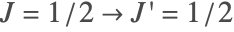system, such as described in [Auzinsh2004]. The pump light is circularly polarized, propagating in thedirection; precession occurs around a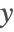-directed magnetic field; optical rotation is measured in probe light propagating alongand linearly polarized along. We calculate the optical rotation assuming a separated pumpprobe arrangement. We then show that a simpler solution can be obtained by further separating the optical rotation process into three stages: pump, precession, and probe.

## Pump-Probe Calculation

 Set DensityMatrix options to consider the steady state.
 We consider a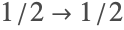transition.
 The pump light is circularly polarized, propagating in thedirection.
 The pump-region Hamiltonian includes the pump field and a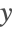-directed magnetic field.
 Apply the rotating-wave approximation to the Hamiltonian.
 The matrix describing relaxation due to atom transit and spontaneous decay.
 The corresponding repopulation matrix.
 Find the density-matrix evolution equations.

The pump-region equations are too complicated to allow a convenient analytical solution, so we will solve them numerically after finding the formula for the optical rotation in the probe region in terms of the ground-state pump-region density matrix.

 The probe light is propagating along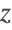and linearly polarized along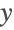.
 The probe-region Hamiltonian; no magnetic field is present.
 Apply the rotating-wave approximation to the probe-region Hamiltonian.

The probe-region relaxation matrix is the same as that for the pump region. The repopulation matrix, on the other hand is different. The probe-region is repopulated by atoms traveling from the pump region, so the transit repopulation matrix is the pump-region ground-state density matrix times the transit rate. We can ignore repopulation due to spontaneous decay in the probe region, since we will solve the probe-region equations to first order only.

 Write the ground-state pump-region density matrix; we distinguish the pump-region density-matrix elements from those of the probe-region by labeling them.
 Find the probe-region evolution equations; the density matrix is repopulated by the pump-region ground-state density matrix at the transit rate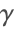.
 The list of density-matrix variables.
 Solve the probe-region equations to first order in the Rabi frequency, in terms of the pump-region ground-state density-matrix elements.
 Find the optical rotation of the probe light, in terms of the probe-region density-matrix elements; note that the parameters supplied to the Observables function must match those of the probe field.
 Substitute the solution for the probe-region density matrix into the rotation formula to find the optical rotation of weak probe light in terms of the pump-region ground state density-matrix elements.
 Solve the pump-region equations numerically as a function of the Larmor frequency and substitute into the rotation formula.

## Pump–Precession–Probe Calculation

The mechanism of optical rotation can be further simplified conceptually and computationally by separating the pump and precession steps (or regions) in order to model a three-stage (pumpprecessionprobe) process.

 The pump-region Hamiltonian is as before, but without the magnetic field.
 Apply the RWA to the pump-region Hamiltonian.
 Find the density-matrix evolution equations, using the same relaxation and repopulation matrices as above.
 Solve the pump-region equations to second order in the Rabi frequency (first order in the light power).
 The precession-region Hamiltonian has only the magnetic field.
 Find the precession-region evolution equations, assuming that the precession region is repopulated by ground-state atoms from the pump region.
 Solve the precession-region equations.
 Substitute the precession-region solution into the perturbative formula for optical rotation and then substitute the pump-region solution into that to find the optical rotation in the probe region.
 Plot the optical rotation as a function of Larmor frequency.

### References

[Auzinsh2004] M. Auzinsh, D. Budker, D.F. Kimball, S.M. Rochester, J.E. Stalnaker, A.O. Sushkov, V.V. Yashchuk, "Can a Quantum Nondemolition Measurement Improve the Sensitivity of an Atomic Magnetometer?," Phys. Rev. Lett. 93, 173002 (2004). https://doi.org/10.1103/PhysRevLett.93.173002.